# Probability Probability theory underlies the statistical hypothesis Slide

• Slides: 26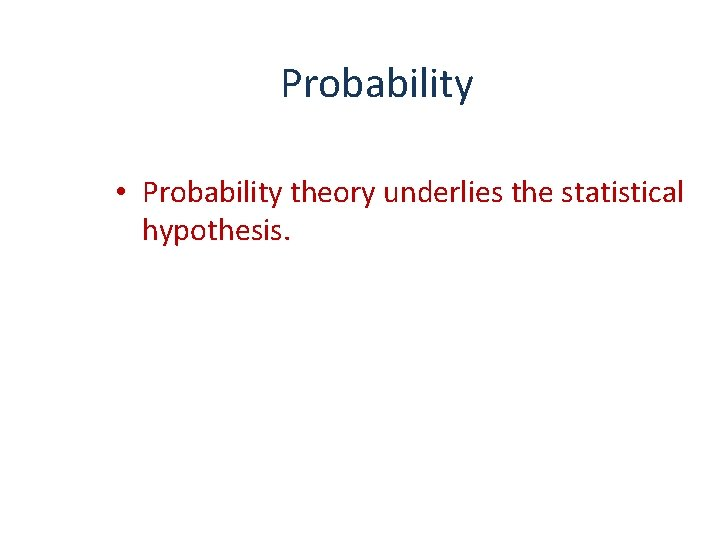Probability • Probability theory underlies the statistical hypothesis. Slide 1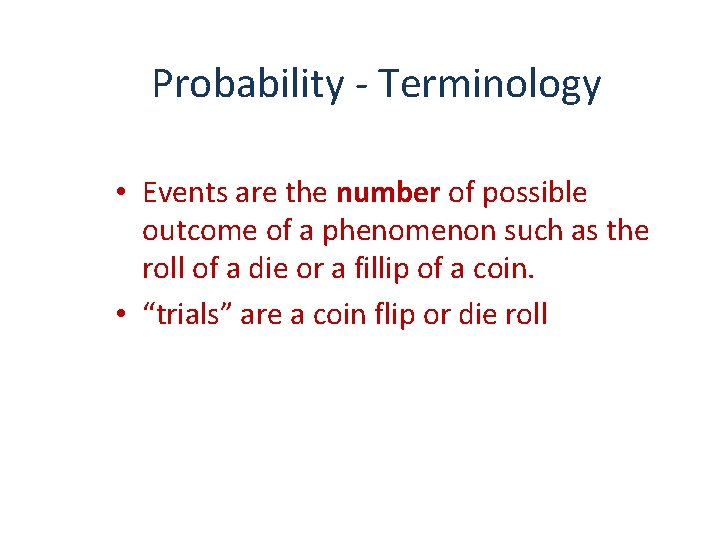Probability - Terminology • Events are the number of possible outcome of a phenomenon such as the roll of a die or a fillip of a coin. • “trials” are a coin flip or die roll Slide 2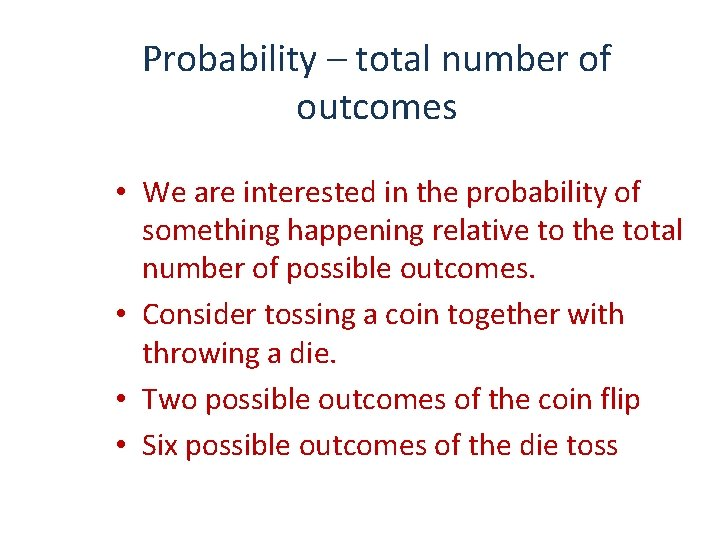Probability – total number of outcomes • We are interested in the probability of something happening relative to the total number of possible outcomes. • Consider tossing a coin together with throwing a die. • Two possible outcomes of the coin flip • Six possible outcomes of the die toss Slide 3Probability - total number of outcomes • Two possible outcomes of the coin flip • Six possible outcomes of the die toss • Without regard for order, what are the total number of outcomes of the two events together? • H 1, H 2, H 3 … Slide 4Probability - total number of outcomes • • Slide 5 Number of events for each trial k 1 = 2, k 2 = 6 So, in general: The number of events = k 1 x k 3 x … kn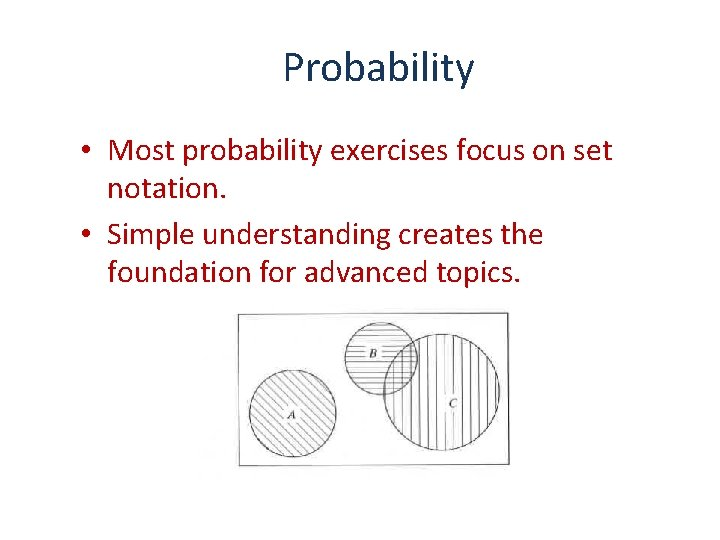Probability • Most probability exercises focus on set notation. • Simple understanding creates the foundation for advanced topics.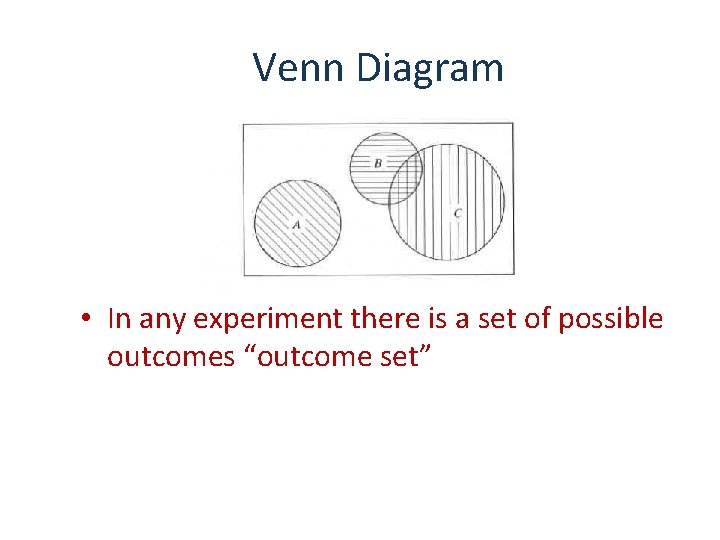Venn Diagram • In any experiment there is a set of possible outcomes “outcome set”Venn Diagram • Intersect – the common elements in two sets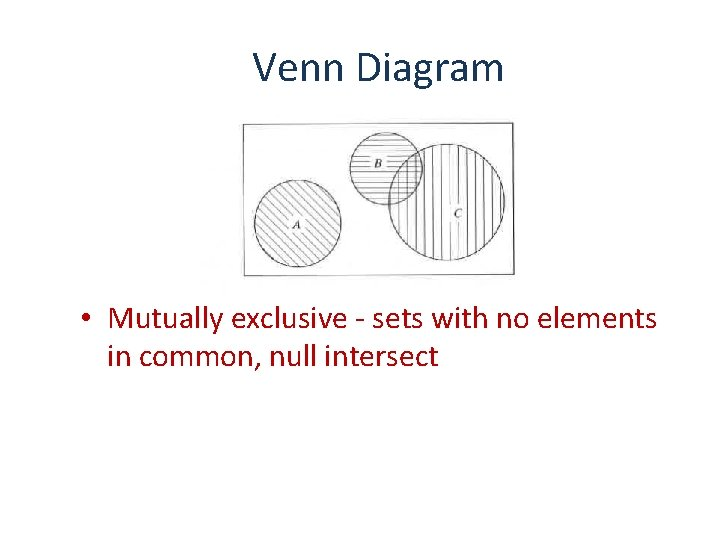Venn Diagram • Mutually exclusive - sets with no elements in common, null intersect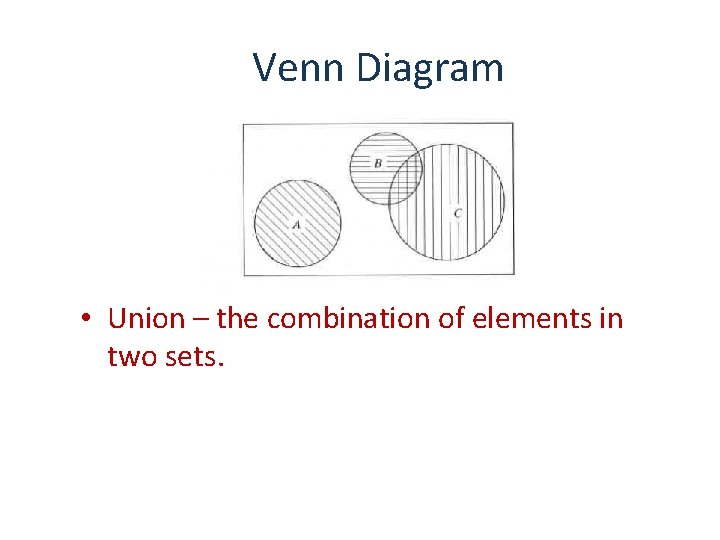Venn Diagram • Union – the combination of elements in two sets.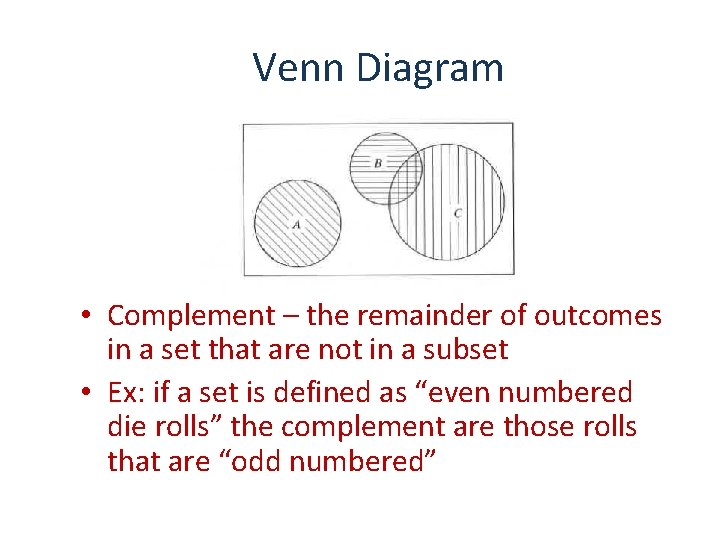Venn Diagram • Complement – the remainder of outcomes in a set that are not in a subset • Ex: if a set is defined as “even numbered die rolls” the complement are those rolls that are “odd numbered”Probability of an event • Define the relative frequency: – N is the total number of events – f is the number of times that outcomes in the subset were observed.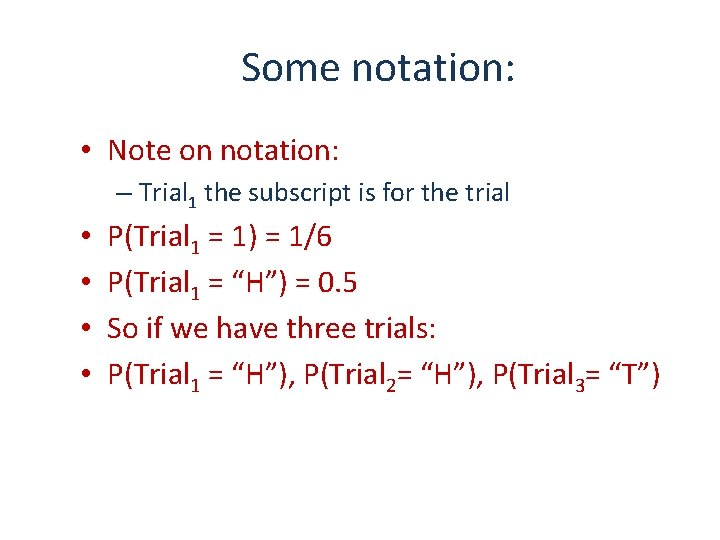Some notation: • Note on notation: – Trial 1 the subscript is for the trial • • P(Trial 1 = 1) = 1/6 P(Trial 1 = “H”) = 0. 5 So if we have three trials: P(Trial 1 = “H”), P(Trial 2= “H”), P(Trial 3= “T”)Mutually exclusive events • If two events are mutually exclusive “A” and “B” then the probability of either event is the sum of the probabilities: • P(A or B) = P(A) + P(B) • P(“HRC win” or “DJT win”) = P(“HRC win”) + “DJT win” • These are disjoint sets – both outcomes cannot be realized.Non-mutually exclusive events • If events are not mutually exclusive: – i. e. . both outcomes can be realized • Sets “Mammals” and “flying vertebrates” are not disjoint. • P(A or B) = P(A) + P(B) – P(A and B)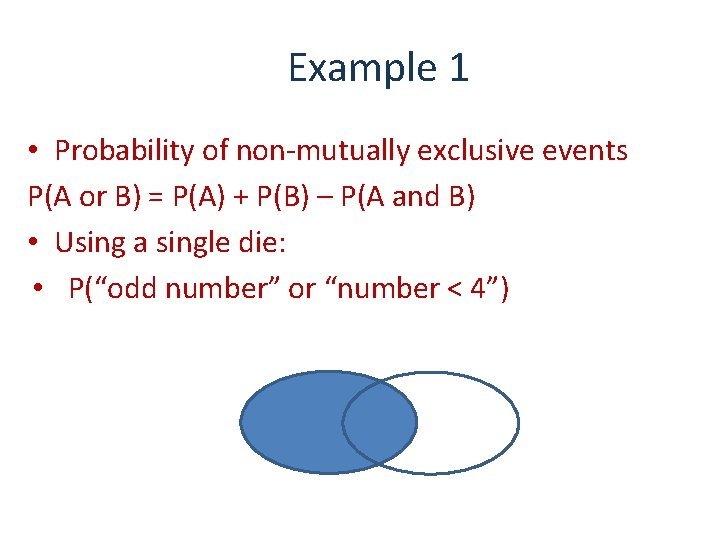Example 1 • Probability of non-mutually exclusive events P(A or B) = P(A) + P(B) – P(A and B) • Using a single die: • P(“odd number” or “number < 4”)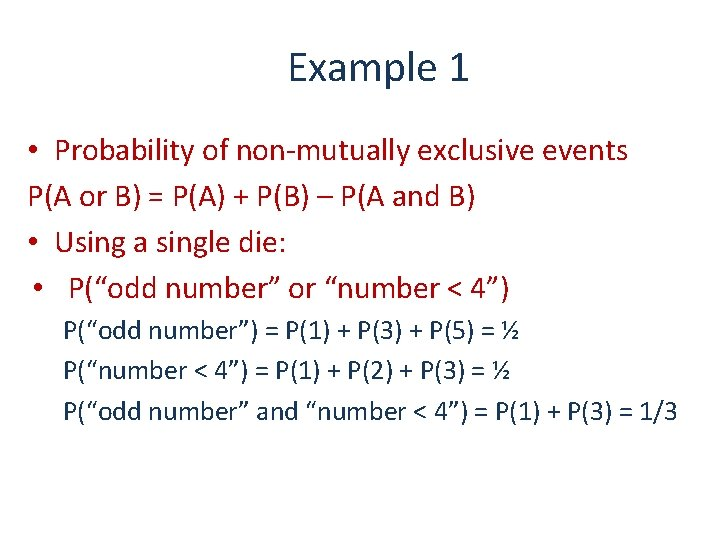Example 1 • Probability of non-mutually exclusive events P(A or B) = P(A) + P(B) – P(A and B) • Using a single die: • P(“odd number” or “number < 4”) P(“odd number”) = P(1) + P(3) + P(5) = ½ P(“number < 4”) = P(1) + P(2) + P(3) = ½ P(“odd number” and “number < 4”) = P(1) + P(3) = 1/3Probability of Events • Mutually exclusive: • P(A or B) = P(A) + P(B) • Non-mutually exclusive: • P(A or B) = P(A) + P(B) – P(A and B)Example 2 • • • Deck of cards 13 in each suit (4 suits) P(“Diamond”) = P(“King”) = P(“Diamond” or “King”) =Example 2 P(A or B) = P(A) + P(B) – P(A and B) • • • Deck of cards 13 in each suit (4 suits) Cards in each suit: 2 to 10, J, Q, K, A P(“Diamond”) = P(“King”) = P(“Diamond” or “King”) =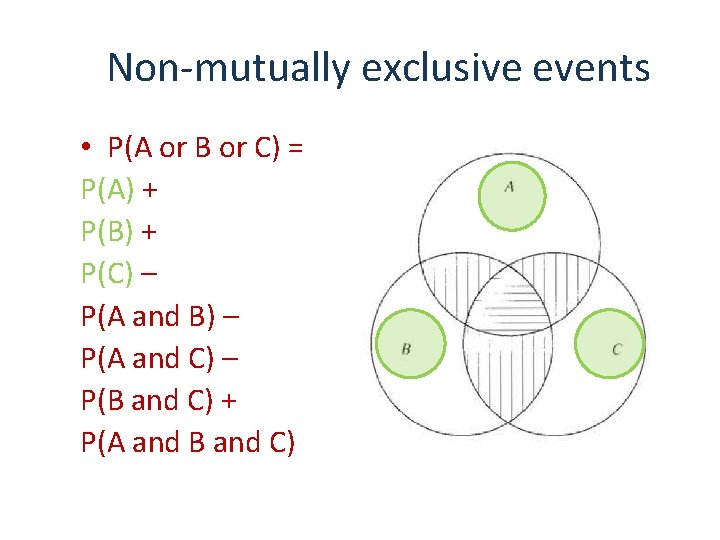Non-mutually exclusive events • P(A or B or C) = P(A) + P(B) + P(C) – P(A and B) – P(A and C) – P(B and C) + P(A and B and C)Non-mutually exclusive events • P(A or B or C) = P(A) + P(B) + P(C) – P(A and B) – P(A and C) – P(B and C) + P(A and B and C)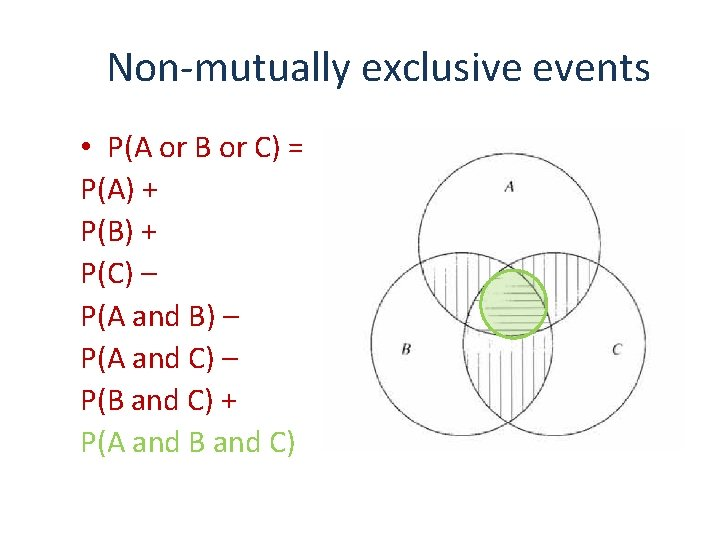Non-mutually exclusive events • P(A or B or C) = P(A) + P(B) + P(C) – P(A and B) – P(A and C) – P(B and C) + P(A and B and C)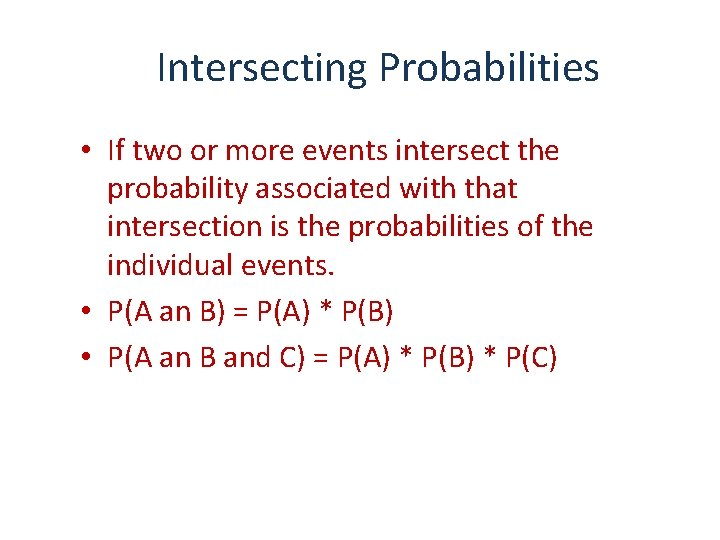Intersecting Probabilities • If two or more events intersect the probability associated with that intersection is the probabilities of the individual events. • P(A an B) = P(A) * P(B) • P(A an B and C) = P(A) * P(B) * P(C)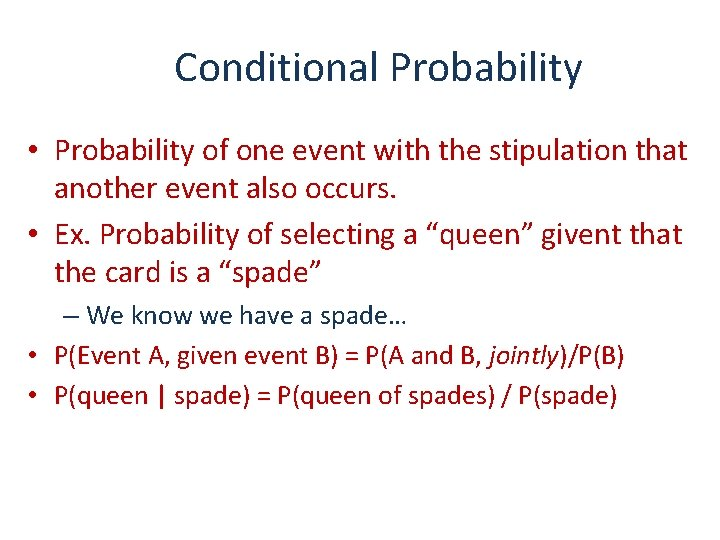Conditional Probability • Probability of one event with the stipulation that another event also occurs. • Ex. Probability of selecting a “queen” givent that the card is a “spade” – We know we have a spade… • P(Event A, given event B) = P(A and B, jointly)/P(B) • P(queen | spade) = P(queen of spades) / P(spade)Conditional Probability, cont’d • P(Event A, given event B) = P(A and B, jointly)/P(B) • P(queen | spade) = P(queen of spades) / P(spade) • P(queen | spade) = frequency of queen of spades/ frequency of spades • Note that this conditional probability is quite different from the probability of selecting a spade, given that the card is a queen… • P(spade | queen) =